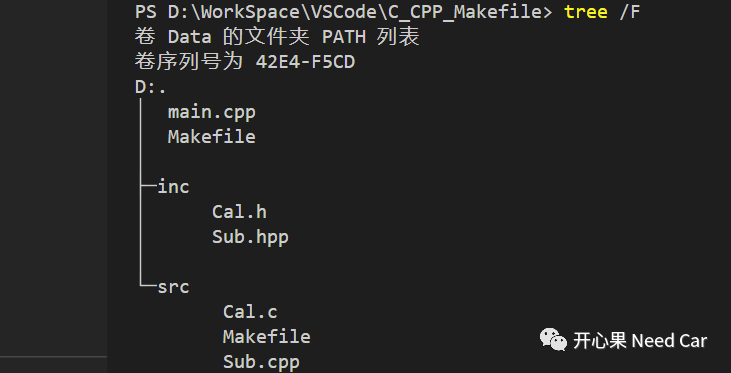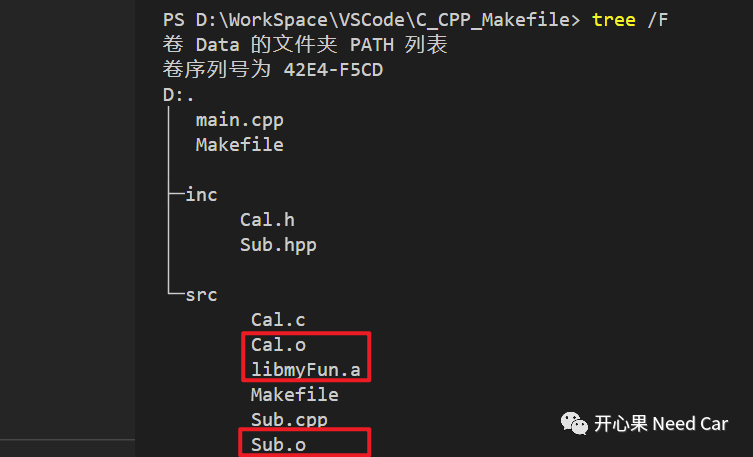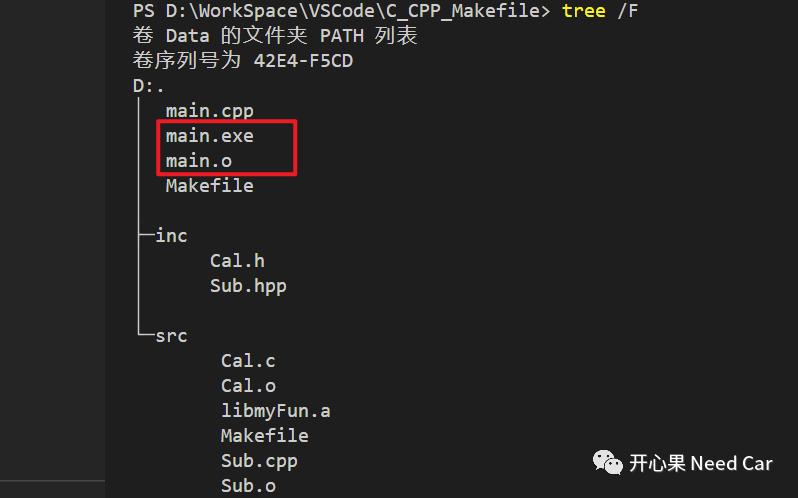# 基于Makefile的C、C++混编

0收藏

1. 如何基于makefile混合编译C、C++

2. 如何生成静态链接库（*.a）

3. 如何使用静态链接库。

### 1、如何基于makefile混合编译C、C++

（一）示例Cal.h文件内容如下所示：

`````` #ifndef _CAL_H_
#define _CAL_H_

typedef int(*calOpt)(int, int);

#ifdef __cplusplus
extern "C"
{
#endif

#ifdef __cplusplus
}
#endif

#endif``````

Sub.hpp文件内容如下所示：

``````#ifndef _SUB_HPP_
#define _SUB_HPP_

extern int sub(int x,int y);

#endif``````

Cal.c文件内容如下所示：

`````` #include "../inc/Cal.h"

{
return (x + y);
}``````

Sub.cpp文件内容如下所示：

`````` #include "../inc/Sub.hpp"

int sub(int x,int y)
{
if( x >= y)
{
return (x - y);
}
else
{
return (y - x);
}
}``````

src文件夹下的Makefile文件内容如下所示：

`````` #指定编译器路径
CROSS_COMPILE = D:/App/Work/Mingw64/mingw64/bin/
#汇编器
AS    = \$(CROSS_COMPILE)as
#链接器
LD    = \$(CROSS_COMPILE)ld
CXX   = \$(CROSS_COMPILE)g++
CC    = \$(CROSS_COMPILE)gcc
#用于创建，修改和提取档案的实用程序
AR    = \$(CROSS_COMPILE)ar
#列出目标文件中的符号
NM    = \$(CROSS_COMPILE)nm
#丢弃的符号
STRIP    = \$(CROSS_COMPILE)strip
#复制并转换目标文件
OBJCOPY    = \$(CROSS_COMPILE)objcopy
#显示目标文件中的信息
OBJDUMP    = \$(CROSS_COMPILE)objdump
export AS LD CXX CC AR NM
export STRIP OBJCOPY OBJDUMP

CFLAGS +=-I. -g -O0 -Wall -fpermissive

#头文件搜索路径
INCLUDE := \
-I ../ \
-I ../inc/
#查找当前路径下所有后缀为.c的文件,保存到SRC_C变量中
SRC_C=\$(wildcard *.c)
#查找当前路径下所有后缀为.cpp的文件,保存到SRC_CPP变量中
SRC_CPP=\$(wildcard *.cpp)
#将SRC_C变量中.c替换为.o并保存到APP_C_OBJS变量中
APP_C_OBJS=\$(SRC_C:.c=.o)
APP_CPP_OBJS=\$(SRC_CPP:.cpp=.o)

#生成静态库libmyFun.a需要依赖中间产物APP_C_OBJS和APP_CPP_OBJS
libmyFun.a:\$(APP_C_OBJS) \$(APP_CPP_OBJS)
\$(AR) rcs \$@ \$^

%.o:%.c
@\$(CC) \$(INCLUDE) -fPIC -c \$< -o \$@ \$(CFLAGS)
%.o:%.cpp
@\$(CXX) \$(INCLUDE) -fPIC -c \$< -o \$@ \$(CPPFLAGS)
clean:
rm -rf *.o *.a``````

main.cpp文件内容如下所示：

`````` #include <iostream>
#include "inc/Cal.h"
#include "inc/Sub.hpp"

int main(){

int result;
result = optFunc(0x10, 0x20);

std::cout << "add result = " << result << std::endl;

/* 通过函数指针调用sub函数 */
optFunc = sub;
result = optFunc(0x10, 0x20);

std::cout << "sub result = " << result << std::endl;
return 0;
}``````

`````` #指定编译器路径
CROSS_COMPILE = D:/App/Work/Mingw64/mingw64/bin/
#汇编器
AS    = \$(CROSS_COMPILE)as
#链接器
LD    = \$(CROSS_COMPILE)ld
CXX   = \$(CROSS_COMPILE)g++
CC    = \$(CROSS_COMPILE)gcc
#用于创建，修改和提取档案的实用程序
AR    = \$(CROSS_COMPILE)ar
#列出目标文件中的符号
NM    = \$(CROSS_COMPILE)nm
#丢弃的符号
STRIP    = \$(CROSS_COMPILE)strip
#复制并转换目标文件
OBJCOPY    = \$(CROSS_COMPILE)objcopy
#显示目标文件中的信息
OBJDUMP    = \$(CROSS_COMPILE)objdump
export AS LD CXX CC AR NM
export STRIP OBJCOPY OBJDUMP

LIB_FLAGS += -L./src\
-l:libmyFun.a
#头文件搜索路径
INCLUDE := \
-I ./ \
-I ./inc/
#查找当前路径下所有后缀为.cpp的文件,保存到SRC_CPP变量中
SRC_CPP=\$(wildcard *.cpp)
#将SRC_CPP变量中.cpp替换为.o并保存到APP_CPP_OBJS变量中
APP_CPP_OBJS=\$(SRC_CPP:.cpp=.o)

TARGETS = main

all: \$(TARGETS)
main: \$(APP_CPP_OBJS)
\$(CXX) \$(LIB_FLAGS) \$(CPPFLAGS) \$(INCLUDE) -o \$(TARGETS) -g \$(APP_CPP_OBJS) -lmyFun
%.o:%.cpp
\$(CXX) \$(INCLUDE) -fPIC -c \$< -o \$@ \$(CPPFLAGS)
clean:
rm -rf *.o \$(TARGETS)``````

提示：每行命令之前使用TAB隔开不是空格

### 2、如何生成静态链接库（*.a）`````` #生成静态库libmyFun.a需要依赖中间产物APP_C_OBJS和APP_CPP_OBJS
libmyFun.a:\$(APP_C_OBJS) \$(APP_CPP_OBJS)
\$(AR) rcs \$@ \$^``````

### 3、如何使用静态链接库